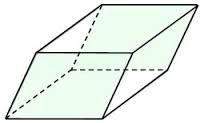# Circumference 67334

What is the area and circumference of a diamond whose diagonals are 7 dm and 12dm in length and 6 dm in height?

S =  42 cm2
o =  28 dm

### Step-by-step explanation:Did you find an error or inaccuracy? Feel free to write us. Thank you!

Tips for related online calculators
Need help calculating sum, simplifying, or multiplying fractions? Try our fraction calculator.

#### Grade of the word problem:

We encourage you to watch this tutorial video on this math problem: